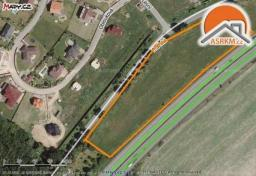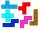# Parcel

parcel has a rectangular shape of a trapezoid with bases 12 m and 10 m and a height 8 m. On parcel was built object with a footprint an isosceles triangle shape with side 4 m and height three-quarters of a meter. What is the area of unbuild parcel?

S =  86.5 m2

### Step-by-step explanation:Did you find an error or inaccuracy? Feel free to write us. Thank you!Tips to related online calculators

#### You need to know the following knowledge to solve this word math problem:

We encourage you to watch this tutorial video on this math problem:

## Related math problems and questions:

• EmbankmentPerpendicular cross-section of the embankment around the lake has the shape of an isosceles trapezoid. Calculate the perpendicular cross-section, where bank is 4 m high the upper width is 7 m and the legs are 10 m long.
• Isosceles trapezoidCalculate the area of an isosceles trapezoid whose bases are in the ratio of 4:3; leg b = 13 cm and height = 12 cm.
• The gardenThe garden has the shape of an isosceles trapezoid whose bases are 64 m and 24 m long, the height is 25 m. On what area of the garden is it possible to grow vegetables if a fifth of the area is occupied by a cottage, lawn and path?
• Trapezoid ABCD v2Trapezoid ABCD has a length of bases in ratio 3:10. The area of triangle ACD is 825 dm2. What is the area of trapezoid ABCD?
• Isosceles trapezoidFind the area of an isosceles trapezoid with bases of 8cm and 72mm. The height of the trapezoid is equal to three-quarters of the longer base.
• Roof tilesThe roof has a trapezoidal shape with bases of 15 m and 10 m, height of roof is 4 meters. How many tiles will need if on 1 m2 should be used 8 tiles?
• Land - isosceles trapezoidCalculate the building plot's content and perimeter in the form of an isosceles trapezoid with bases 120m, 95m, and height 50m.
• Trapezoid MO-5-Z8Trapezoid KLMN has bases 12 and 4 cm long. The area of triangle KMN is 9 cm2. What is the area of the trapezoid KLMN?
• EstateAn estate-shaped rectangular trapezoid has bases long 34 m, 63 m, and perpendicular arm 37 m. Calculate how long its fence is.Road embankment has a cross-section shape of an isosceles trapezoid with bases 5 m and 7 m, and 2 m long leg. How many cubic meters of soil is in embankment length of 1474 meters?
• Isosceles trapezoidThe lengths of the bases of the isosceles trapezoid are in the ratio 5:3, the arms have a length of 5 cm and height = 4.8 cm. Calculate the circumference and area of a trapezoid.
• Isosceles trapezoidCalculate the circumference and the contents of the isosceles trapezoid if you know the size of the bases is 8 and 12 cm and the size of the arms is 5 cm.
• Isosceles trapezoidFind the area of an isosceles trapezoid, if the bases are 12 cm and 20 cm, the length of the arm is 16 cm
• Railway embankmentThe railway embankment section is an isosceles trapezoid, the sizes of the bases of which are in the ratio 5: 3. The arms have a length of 5 m, and the height of the embankment is 4.8 m. Calculates the size of the embankment section area.
• The basesThe bases of the isosceles trapezoid ABCD have lengths of 10 cm and 6 cm. Its arms form an angle α = 50˚ with a longer base. Calculate the circumference and content of the ABCD trapezoid.
• Isosceles trapezoidCalculate the content of an isosceles trapezoid whose bases are at ratio 5:3, the arm is 6cm long and it is 4cm high.
• LegDetermine the trapezoid area with bases 71 and 42, and the height is 4 shorter than its leg.